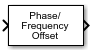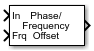Phase/Frequency Offset

Apply phase and frequency offsets to complex baseband signal

• Library:
• Communications Toolbox / RF Impairments Correction

Communications Toolbox / RF Impairments

•Description

The Phase/Frequency Offset block applies phase and frequency offsets to a complex signal.

This icon shows the block with all ports enabled.Ports

Input

expand all

Complex signal, specified as a scalar, vector, or matrix. The port is unnamed until you enable the Frequency offset from port parameter.

Data Types: double | single
Complex Number Support: Yes

Frequency offset, specified as a scalar, a vector with the same number of rows or columns as the input signal, or a matrix with the same dimensions as the input signal. For more information, see Interdependent Parameter-Port Dimensions.

Dependencies

To enable this port, select the Frequency offset from port parameter.

Data Types: double | single

Output

expand all

Output signal, returned as a scalar, vector, or matrix. This output is the same dimension and data type as the input signal.

Parameters

expand all

Phase offset in degrees, specified as a scalar, vector, or matrix.

If Phase offset (deg) and Frequency offset (Hz) are both nonscalar, they must be the same size.

Tunable: Yes

Select this parameter to add the Frq port.

• When you select this parameter, the Frq port specifies the frequency offset.

• When you clear this parameter, the Frequency offset (Hz) parameter specifies the frequency offset.

Frequency offset in hertz, specified as a scalar, a vector with the same number of rows or columns as the input signal, or a matrix with the same dimensions as the input signal. For more information, see Interdependent Parameter-Port Dimensions.

If Phase offset (deg) and Frequency offset (Hz) are both nonscalar, they must be the same size.

Tunable: Yes

Dependencies

To enable this port, clear the Frequency offset from port parameter.

Block Characteristics

 Data Types double | single Multidimensional Signals no Variable-Size Signals no

expand all

Algorithms

If the input signal is u(t), then the output signal is

$y\left(t\right)=u\left(t\right)\cdot \left(\mathrm{cos}\left(2\pi {\int }_{0}^{t}f\left(\tau \right)d\tau +\phi \left(t\right)\right)+j\mathrm{sin}\left(2\pi {\int }_{0}^{t}f\left(\tau \right)d\tau +\phi \left(t\right)\right)\right)\text{,}$

where f(t) is the frequency offset, and φ(t) is the phase offset.

The discrete-time output is given by

where i > 0, and Δt is the sample time.

Extended Capabilities

Introduced before R2006a